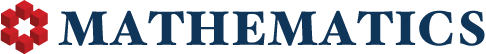1

(click on this box to dismiss)

Displays the number of upvotes and downvotes received on questions and answers and their ratio for the top 1000 users by reputation. Note that 999999999 represents a user with no downvotes.Q&A for people studying math at any level and professionals in related fields

``````-- Ratio of Upvotes to Downvotes Received.
-- Displays the number of upvotes and downvotes received on questions and answers and their ratio for the top 1000 users by reputation. Note that 999999999 represents a user with no downvotes.
select
SUM(CASE WHEN v.VoteTypeId = 2 THEN 1 ELSE 0 END) AS [Up Votes],
SUM(CASE WHEN v.VoteTypeId = 3 THEN 1 ELSE 0 END) AS [Down Votes],
case when SUM(CASE WHEN v.VoteTypeId = 3 THEN 1 ELSE 0 END) = 0 then 999999999 else
(CAST(SUM(CASE WHEN v.VoteTypeId = 2 THEN 1 ELSE 0 END) AS float) /
CAST(SUM(CASE WHEN v.VoteTypeId = 3 THEN 1 ELSE 0 END) AS float)) end
u.DisplayName
fromHold tight while we fetch your results